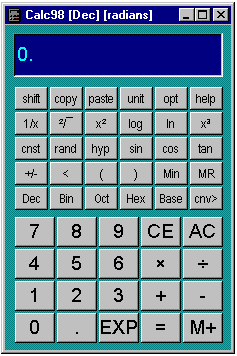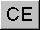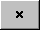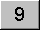calculator.org

# Calculator BasicsAs you would expect, you use the calculator just as you would a real pocket calculator. Just as with a conventional calculator, space is at a premium, so many of the calculator buttons have to double up the functions they provide. The layout of the calculator in its normal state has the functions you would expect to see on a fairly standard scientific calculator, which means that it is probably self explanatory. Notice the shift button in the top left hand corner.

Enter numbers either by clicking on the buttons or using the keyboard (note: to use a numeric keypad make sure Num Lock is on). To enter a number in exponential format, enter the mantissa, followed by the EXP key, then +/- if required, then the exponent. To use the arithmetic functions, click their buttons. If the function is unary (i.e. acts on one number, e.g. 1/x) the display is updated to the result. If the function is binary (e.g. +, -, X etc.) enter the second number and click = or another arithmetic key.

The functions of the buttons in their shifted state are not marked on the calculator body, but the buttons change when you press the shift button. If you press the shift button again, the buttons revert to their un-shifted state.

Most of the calculator buttons can be accessed from your computer keyboard (these are sometimes called 'keyboard shortcuts' or 'keyboard accelerators'). Keyboard accelerators are listed by key and by meaning. You can also use the Caps Lock key on your keyboard instead of the shift button.

An optional menu bar (or command bar) can be used to give access to additional functions. All the menu bar functions can be obtained in other ways. Activate the menu bar using the Layout tab of the Options dialog.

##- All Clear

Clears the calculator, and resets any functions. The contents of the memory are not affected by AC. The memory can be cleared after pressing AC by pressing Min.

##- Clear Entry

Erases the last number or operation entered.

##- Minus

This button performs subtraction.

##- Divide

This button performs division.

##- Multiply

This button performs multiplication.

##- Equals

Displays the result of an arithmetic operation. It can be used during a calculation to ensure that an intermediate result has been evaluated. Unless the = button is pressed, it is possible that an operation is incomplete - pressing = ensures that no arithmetic operations are pending.

Certain functions make use of the = button to signify the end of input, in order to evaluate a result.

##- Percent key

This button (or Enter button) displays the result of an arithmetic operation as a percentage.

Examples:
Calculate five sixteenths as a percentage.

5 / 1 6 shift %
Result: 31.25%

Calculate 17.5% of £ 250.00:
250 x 17.5 shift %
Result: £ 43.75

Calculate 12% mark-up on \$250
250 + 12 shift %
Result: \$280

Give 5% discount on goods costing \$125
125 - 5 shift %
Result: \$118.75

##- Pi key

This button (shifted Exp button) displays the value of pi (the ratio of the circumference of a circle to its diameter).

##- Memory Plus

Pressing this button adds the number displayed to the contents of the memory. Additional memories can be accessed by pressing the shift key before pressing this button, which will change to Mn+.

##- Memory n Plus

Add to one of ten memories (labelled 0-9) using this button, which is obtained by pressing the shift button. After pressing Mn+ press the number button corresponding to the desired memory. Memory number zero is the default memory (i.e. the memory referred to by the un-shifted memory buttons).

##- Memory Input

Press the min button to enter the currently displayed number into the memory.

If you first press shift before Min you can select one of the ten memories (0-9). Memory zero is the default memory, i.e. the memory available without pressing the shift button.

##- Memory n Input

Input a value to one of ten memories (labelled 0-9) using this button, which is obtained by pressing the shift button. After pressing MnIn press the number button corresponding to the desired memory. Memory number zero is the default memory (i.e. the memory referred to by the un-shifted memory buttons). The "Clear All" button allows you to delete the contents of all the memories.

##- Memory Recall

Use this button to put the contents of the memory onto the display. The default memory is number zero. To display other memories, first press the shift button and then the MR button and select the required memory.

##- Memory n Recall

Access one of ten memories (labelled 0-9) using the this button, which is obtained by pressing the shift button. After pressing MnR press the number button corresponding to the desired memory. Memory number zero is the default memory (i.e. the memory referred to by the un-shifted memory buttons).

##- Decimal Point

Use the point button to enter the decimal part of a number. The decimal point is disabled in non-decimal number systems.

##- Exponent

Use this button to enter a number in exponential form.

Example:
To enter the number 1.234 x 10^6 (1.234E6) press the following buttons:

1 . 2 3 4 EXP 6

##- Number buttons

Number buttons are used in all modes. In Hexadecimal mode they are augmented by the letters A to F. In Octal mode the 8 and 9 buttons are disabled. In Binary mode only the numbers 0 and 1 are enabled. In statistics mode the number buttons are used with the shift button to obtain the statistical functions.

##- Shift

Use to access additional functions. The functions available depend on the calculator mode, and are indicated by changes to the legends on the calculator buttons.

## Errors

If you get an error, the calculator display will contain an appropriate message, and possibly some further descriptive information. In the case of certain errors, the calculator functions are disabled and you need to press the AC button to clear the error.